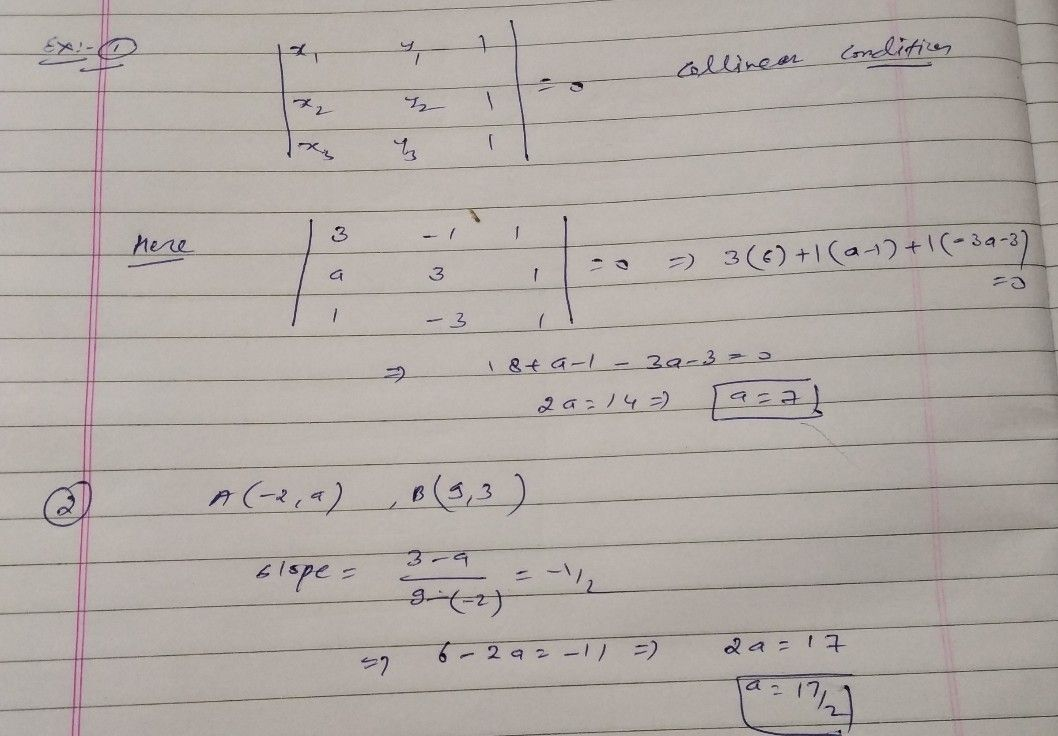Symbol
ProblemIf the three points $\left(3-1\right),\left(a,3\right)$ and $\left(1-3\right)$ are collinear, find the value of a. The line through the points $\left(-2a\right)$ and $\left(9,3\right)$ $h2sslope-\dfrac {1} {2}$ Find the value of $5\right)$ and $\left(4,8\right)$ is perpendicura arou
10th-13th grade
Other
Search count: 106
SolutionQanda teacher - bhagibish4Student
How does the question form?
Sry equation
Excuse me sir
How does the equation form?